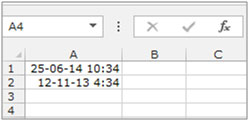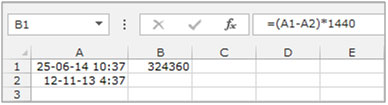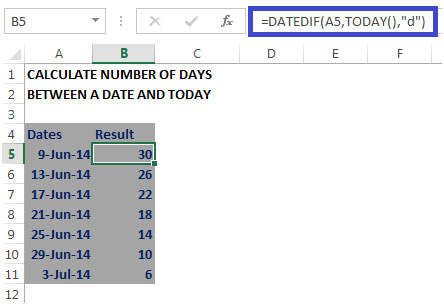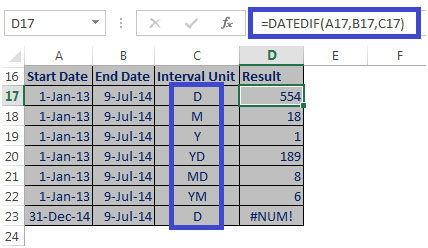Calculate number of minutes between dates & time in Microsoft Excel

In this article we will learn about how to Calculate Number of Minutes between Dates and Time in Microsoft Excel 2010.

To calculate the number of minutes between two dates and times follow the below given steps.

We have two dates with time, 1st date in cell A1 and 2nd date in cellA2.• Select the Cell B1
• Enter the formula = (A1-A2)*1440. In the formula you have to minus the 1st date from the to 2nd date.  That is, you have to minus the small date from the large date. To convert the time into minutes you have to multiply by 1440. (24 hours * 60 minutes = 1440).
• Press enter on the keyboard.
• The function will return 324360 minutes.This value returned is the difference between two dates in terms of the number of minutes between the two.  This number can then be used for other calculations.

Calculate time difference between Date and Today

To calculate number of days, months or years between two dates, we will use Datedif Function.

Column A contains Dates & in column B we want to see the Result.

See example 1 below -

Example1Datedif function is used to calculate the interval between two dates in days, months or years.

Syntax =DATEDIF(start_date, end_date, interval_unit)

The Datedif function has 3 arguments:

• start_date: the starting date
• end_date: the last date
• interval_unit: tells the function to find the number of days (“D”), complete months (“M”), complete years (“Y”), count of days after the current year (“YD”), count of months after the current year (“YM”), count of days after the current month (“MD”) between the two dates.

In example 1, considering that the date the file was created was 9th July 2014, the number of days between 9th June 2014 and the file created date is 30 days which is returned in cell B5 above.  Similarly, the other values are calculated.  Instead of entering the current date / file created date, today() is entered which will keep changing as the date changes.

Example2

In the below screenshot, we have Start Date, End Date & Interval Unit in column A, B & C respectively. The result will be returned in column D.• In cell D17, the Datedif function is calculating the result based on the Interval Unit provided in C17.  Hence, we get the number of days as 554.
• In cell D18, we get 18 as there is a difference of 18 months in between both these dates.
• In cell D19, we get 1 as the result as there is a difference of 1 year between the year of the start date and the year of the end date.
• In cell D20, we get 189 as the result, as we need to exclude the first year and count the number of days from 1-Jan-14 to 9-Jul-14 which is 189.

• Similarly in cell D21, we get 8 as the result, as it is the difference between 9-Jul-2014 and 1-Jul-2014.
• In cell D22, we get 6 as the result.  It counts the number of months after the current year which is 6 months.
• In cell D23,the #NUM! error appears as the start_date is greater than the end_date.  It could also occur if the interval_unit has an invalid value.

1.I have 2 columns with a start time and finish time. However, the columns are both in ‘number’ format (to 2 decimal places). How can I calculate the time difference in minutes? Can anyone help me ?

2.“excele
nt useful tips. thank u .
phani kumar”

3.“Hi Craig,

I will assume that you mean that the columns contain date / time values to two decimal places.

If so, subtract one from the other, and this will give you the time difference in days (Excel’s native unit of time).

You can then format the rsult to display this answer in minutes by using a custom number format such as:

[m]“” mins””

This will *display* the answer in minutes.

Hope that helps,

Alan.”

4.I have done a daily time sheet in excel, now when I try to total the Total worked hours for a month, it just does not seem to work.. can u help

5.“Hi Damien,

First thing I would check is your units.

Are you recording the time in standard Excel units (being days = 24 hours)?

If not, then when you try to add them togather you may get odd results.

Note that you can format the cells to *show* your time in hours – that is a separate issue from what the cells contain though.

That does not mean you cannot use hours or any other units you like, but if you do, you need to be very careful throughout your calculations.

Alan.”

6.“Anyone know the formula for calculating Hrs between start tme and end time to come up with hours worked less 30 minutes for lunch?
ie, Start Time =9:00 A.M End Time =5:30 P.M. This should = 8 hrs.

7.“Anyone know the formula in excel for calculating Hrs between start time and end time to come up with hours worked less 30 minutes for lunch and have the results for each employee you add total up in one cel?
ie,
Employee #1 Start Time in B8=9:00 A.M End Time =5:30 P.M. This should = in B7 8 hrs.
Employee #2 Start Time in B9=9:00 A.M End Time =5:30 P.M. This should = in B7 16 hrs.
Employee #3 Start Time in B10=9:00 A.M End Time =5:30 P.M. This should = in B7 24 hrs.
And so on, as I have 35 employees.

8.“Search for this tip at this web site:

Add time value exceed 24 hours in Microsoft Excel

9.“I don’t see the [m] format in my custom formats.
I am still finding it difficult to establish an hourly rate formula when an operator works (for example) 1:48 and produces 50,000 units. I can tell the system to add 60 + 48 to get 108 minutes and then procede, but how can we calculate if there are 3, 4 or 0 hours plus minutes possible? (IE: 3:06 / 4:42 / 0.35)”

10.“Hi Tom,

If you have the time spent in column A, and the units in column B as follows (A1:B4):

1:48 50000
3:06 85000
4:42 113000
0:35 17000

I am assuming that the times are entered as times (not as text for example).

Then you can calculate the average units per day (being 24 hours) as follows in column C:

=B1/A1

You may have to format the answer to General (for example) since it will probably auto-format as a time.

In this example, C1 = 666666.666… units per day (24 hours).

If you want it per hour, then divide by 24.

If you want it per minute then divide by 1440 (24*60).

Just watch your units and you should be fine.

Alan. “

11.“If you don’t see a custom format already listed, just type it into the box above the list.

Alan.”

12.Need to combine date into one column so that other calculation formulas can handle them for subtraction from other dates & times to yield hours and minutes between two dates. Help—diane

13.“Hi Diane,

Assuming that your two columns (date and time) are already ‘clean’ and are in A1:B4 then you can just add them together:

C1 = A1 + B1

However, for this to work, your dates need to be exact dates, not dates with times (in other words the date values are integers), and the times need to be pure times, with no date element (in other words, the time values need to be greater than or equal to zero, and less than 1).

HTH,

Alan.”

14.“Thank you, Alan, however the tip you shared did not yield the desired outcome.

A B C
1 4/11/2003 07:46
2 4/11/2003 07:46 4/11/03 7:46

I have data as seen in line one, and can not find a formula or method to combine the date & time into ONE column without losing data. In line two here, I show my desired product. End goal is to determine time intervals between different DATES AND TIMES. TQM to the max! desperate!! Anybody???”

15.“Hi Diane,

I just tried it, and it works perfectly for me in Excel 97 and Excel 2000.

You do not indicate *why* or *how* it doesn’t work, but I would gues the most likely cause it that either your dates or times do not meet the criteria I outlined above.

If you clean up the two ‘input’ columns first, does it then work?

Alan.
DATE WORKSHEET AUTOMATICAL
JEFF Posted on: 31-12-1969
WHEN I OPEN THE WORKSHEET I WANT THE WORKSHEET DATED, BUT WHEN I SAVE THE WORKSHEET I DO NOT WANT IT TO CHANGE THE DATE LATER WHEN I OPEN IT AGIAN.

16.I have 2 columns with a start time and finish time. However, the columns are both in ‘number’ format (to 2 decimal places). How can I calculate the time difference in minutes? Can anyone help me ?

17.“excele
nt useful tips. thank u .
phani kumar”

18.“Hi Craig,

I will assume that you mean that the columns contain date / time values to two decimal places.

If so, subtract one from the other, and this will give you the time difference in days (Excel’s native unit of time).

You can then format the rsult to display this answer in minutes by using a custom number format such as:

[m]“” mins””

This will *display* the answer in minutes.

Hope that helps,

Alan.”

19.I have done a daily time sheet in excel, now when I try to total the Total worked hours for a month, it just does not seem to work.. can u help

20.“Hi Damien,

First thing I would check is your units.

Are you recording the time in standard Excel units (being days = 24 hours)?

If not, then when you try to add them togather you may get odd results.

Note that you can format the cells to *show* your time in hours – that is a separate issue from what the cells contain though.

That does not mean you cannot use hours or any other units you like, but if you do, you need to be very careful throughout your calculations.

Alan.”

21.“Anyone know the formula for calculating Hrs between start tme and end time to come up with hours worked less 30 minutes for lunch?
ie, Start Time =9:00 A.M End Time =5:30 P.M. This should = 8 hrs.

22.“Anyone know the formula in excel for calculating Hrs between start time and end time to come up with hours worked less 30 minutes for lunch and have the results for each employee you add total up in one cel?
ie,
Employee #1 Start Time in B8=9:00 A.M End Time =5:30 P.M. This should = in B7 8 hrs.
Employee #2 Start Time in B9=9:00 A.M End Time =5:30 P.M. This should = in B7 16 hrs.
Employee #3 Start Time in B10=9:00 A.M End Time =5:30 P.M. This should = in B7 24 hrs.
And so on, as I have 35 employees.

23.“Search for this tip at this web site:

Add time value exceed 24 hours in Microsoft Excel

24.“I don’t see the [m] format in my custom formats.
I am still finding it difficult to establish an hourly rate formula when an operator works (for example) 1:48 and produces 50,000 units. I can tell the system to add 60 + 48 to get 108 minutes and then procede, but how can we calculate if there are 3, 4 or 0 hours plus minutes possible? (IE: 3:06 / 4:42 / 0.35)”

25.“Hi Tom,

If you have the time spent in column A, and the units in column B as follows (A1:B4):

1:48 50000
3:06 85000
4:42 113000
0:35 17000

I am assuming that the times are entered as times (not as text for example).

Then you can calculate the average units per day (being 24 hours) as follows in column C:

=B1/A1

You may have to format the answer to General (for example) since it will probably auto-format as a time.

In this example, C1 = 666666.666… units per day (24 hours).

If you want it per hour, then divide by 24.

If you want it per minute then divide by 1440 (24*60).

Just watch your units and you should be fine.

Alan.”

26.“If you don’t see a custom format already listed, just type it into the box above the list.

Alan.”

27.Need to combine date into one column so that other calculation formulas can handle them for subtraction from other dates & times to yield hours and minutes between two dates. Help—diane

28.“Hi Diane,

Assuming that your two columns (date and time) are already ‘clean’ and are in A1:B4 then you can just add them together:

C1 = A1 + B1

However, for this to work, your dates need to be exact dates, not dates with times (in other words the date values are integers), and the times need to be pure times, with no date element (in other words, the time values need to be greater than or equal to zero, and less than 1).

HTH,

Alan.”

29.“Thank you, Alan, however the tip you shared did not yield the desired outcome.

A B C
1 4/11/2003 07:46
2 4/11/2003 07:46 4/11/03 7:46

I have data as seen in line one, and can not find a formula or method to combine the date & time into ONE column without losing data. In line two here, I show my desired product. End goal is to determine time intervals between different DATES AND TIMES. TQM to the max! desperate!! Anybody???”

30.“Hi Diane,

I just tried it, and it works perfectly for me in Excel 97 and Excel 2000.

You do not indicate *why* or *how* it doesn’t work, but I would gues the most likely cause it that either your dates or times do not meet the criteria I outlined above.

If you clean up the two ‘input’ columns first, does it then work?

Alan.
DATE WORKSHEET AUTOMATICAL
JEFF Posted on: 31-12-1969
WHEN I OPEN THE WORKSHEET I WANT THE WORKSHEET DATED, BUT WHEN I SAVE THE WORKSHEET I DO NOT WANT IT TO CHANGE THE DATE LATER WHEN I OPEN IT AGIAN.”

31.this iz smhw great

32.I’m trying to find the minutes and seconds calculator but I can’t find it.

Terms and Conditions of use

The applications/code on this site are distributed as is and without warranties or liability. In no event shall the owner of the copyrights, or the authors of the applications/code be liable for any loss of profit, any problems or any damage resulting from the use or evaluation of the applications/code.